# CS 430 Computer Graphics Vectors Part IV Polygon

• Slides: 19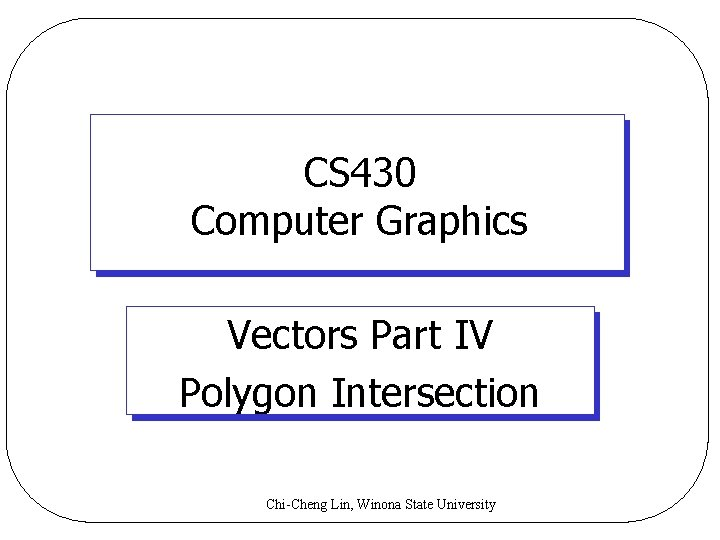CS 430 Computer Graphics Vectors Part IV Polygon Intersection Chi-Cheng Lin, Winona State University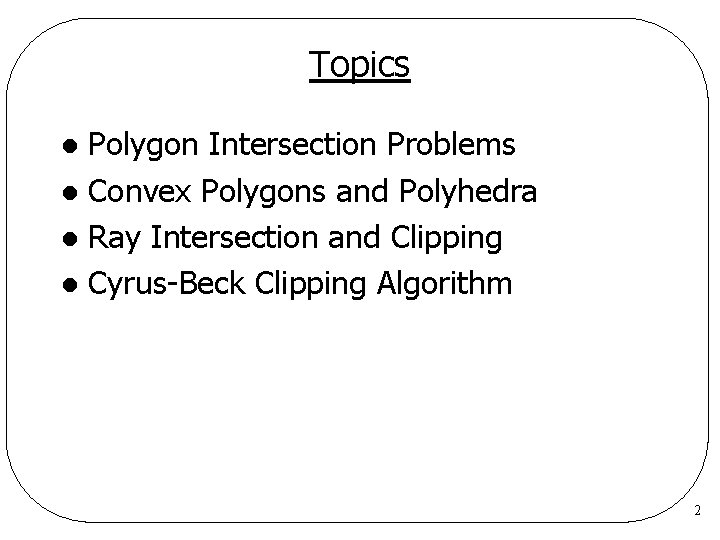Topics Polygon Intersection Problems l Convex Polygons and Polyhedra l Ray Intersection and Clipping l Cyrus-Beck Clipping Algorithm l 2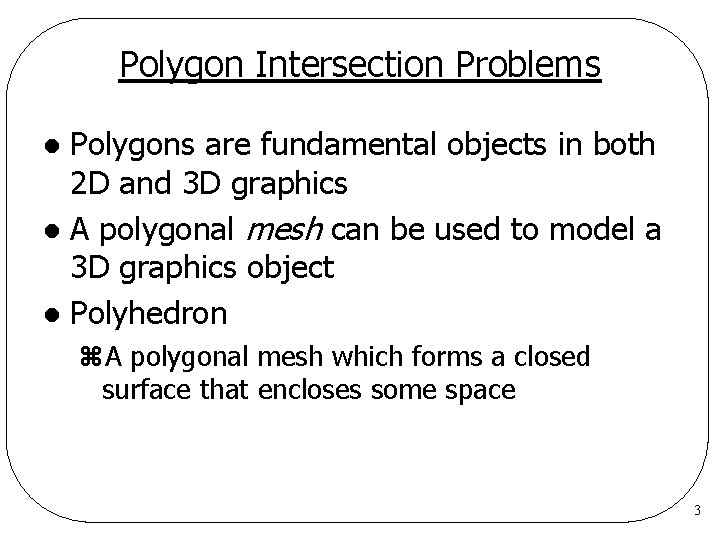Polygon Intersection Problems Polygons are fundamental objects in both 2 D and 3 D graphics l A polygonal mesh can be used to model a 3 D graphics object l Polyhedron l z. A polygonal mesh which forms a closed surface that encloses some space 3Polygon Intersection Problems Is a given point P inside or outside the object? l Where does a given ray R first intersect the object? l Which part of a given line L lies inside/outside the object? l 4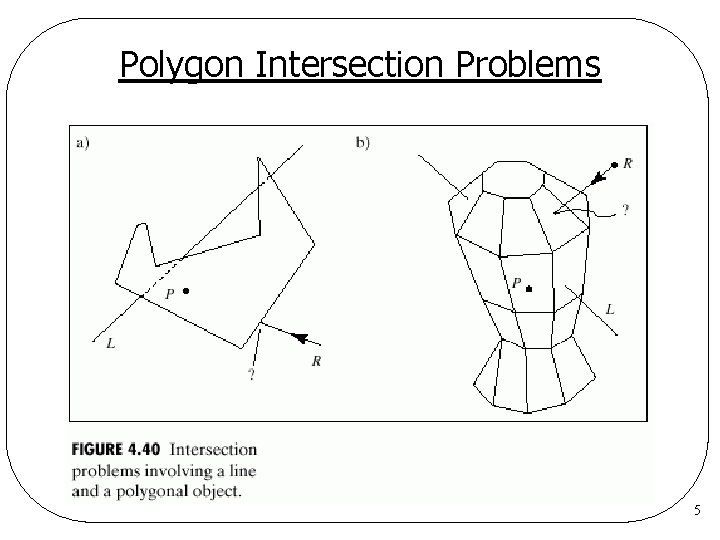Polygon Intersection Problems 5Convex Polygons and Polyhedra General case of polygon/polyhedron intersection problems is complex l Convex polygons/polyhedra are easier to deal with l z 2 D: a convex polygon can be completely described by a set of “bounding lines” z 3 D: a convex polyhedron can be completely described by a set of “bounding planes” Deal with bounding lines/planes 6Convex Polygons and Polyhedra 7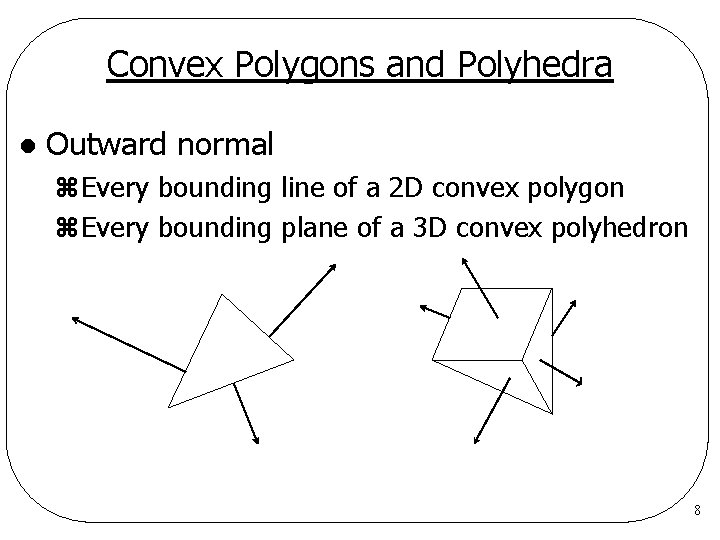Convex Polygons and Polyhedra l Outward normal z. Every bounding line of a 2 D convex polygon z. Every bounding plane of a 3 D convex polyhedron 8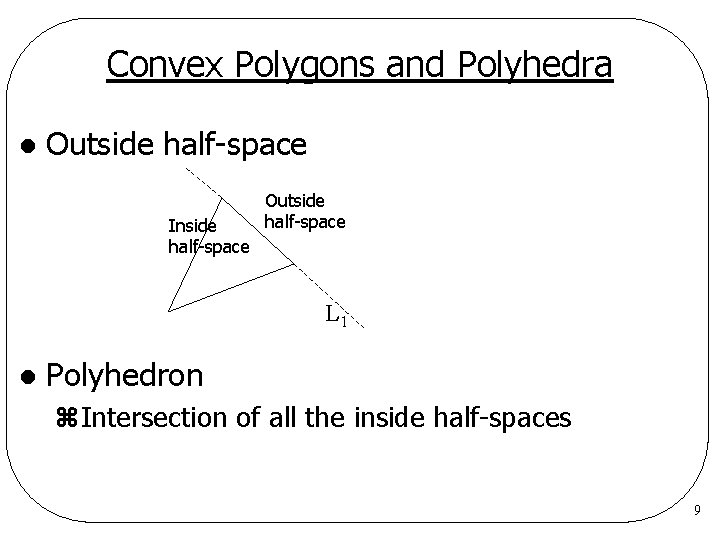Convex Polygons and Polyhedra l Outside half-space Inside half-space Outside half-space L 1 l Polyhedron z. Intersection of all the inside half-spaces 9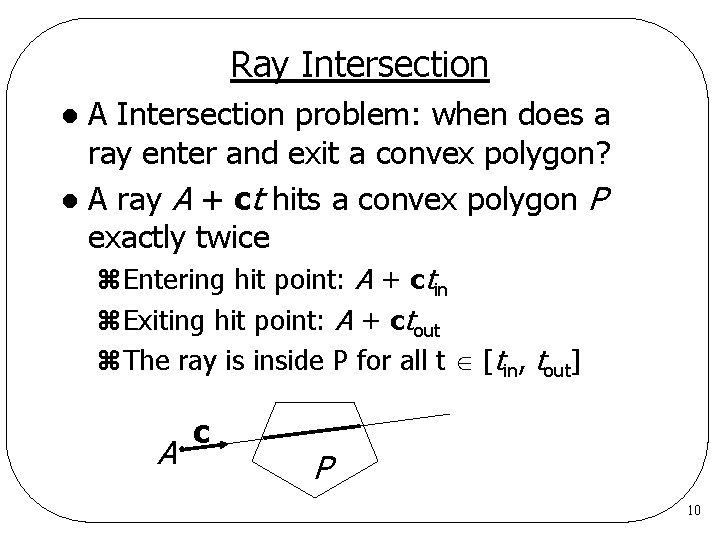Ray Intersection A Intersection problem: when does a ray enter and exit a convex polygon? l A ray A + ct hits a convex polygon P exactly twice l z. Entering hit point: A + ctin z. Exiting hit point: A + ctout z. The ray is inside P for all t [tin, tout] A c P 10Clipping problem: given points A and C, which part of line segment AC lies inside a convex polygon P ? l A’ = A + c max(0, tin) C’ = A + c min(tout, 1) l 0 A tout tin P 1 C 0 1 tout tin C A tin 0 1 tout A C 11Clipping l How are tin and tout computed? z. We must find the intersection of the ray and each bounding line in turn l Assume a bounding line is represented as {B, n}, where z. B: some point on the line zn: outward normal 12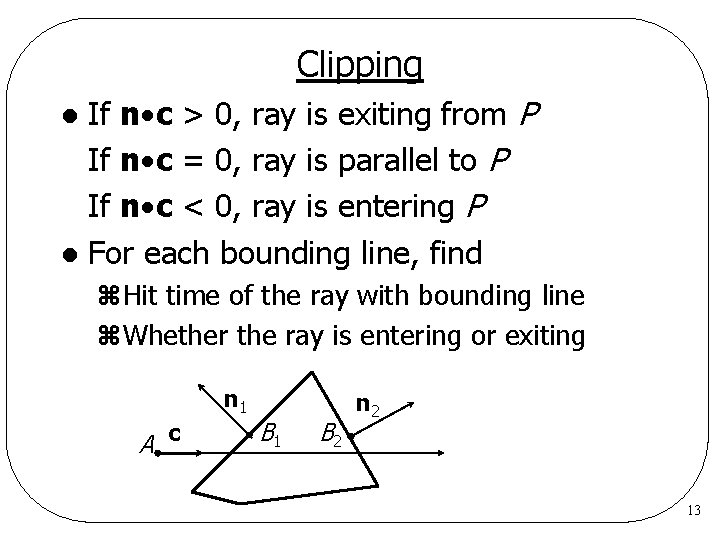Clipping If n c > 0, ray is exiting from P If n c = 0, ray is parallel to P If n c < 0, ray is entering P l For each bounding line, find l z. Hit time of the ray with bounding line z. Whether the ray is entering or exiting n 1 A c B 1 B 2 n 2 13Clipping l Approach z. Candidate interval of t : [tin, tout ] z. Keep track of the maximum entering time tin z. Keep track of the minimum exit time tout z. We want to chop the interval at each iteration z. Can stop as soon as tin > tout (what does this mean? ) 14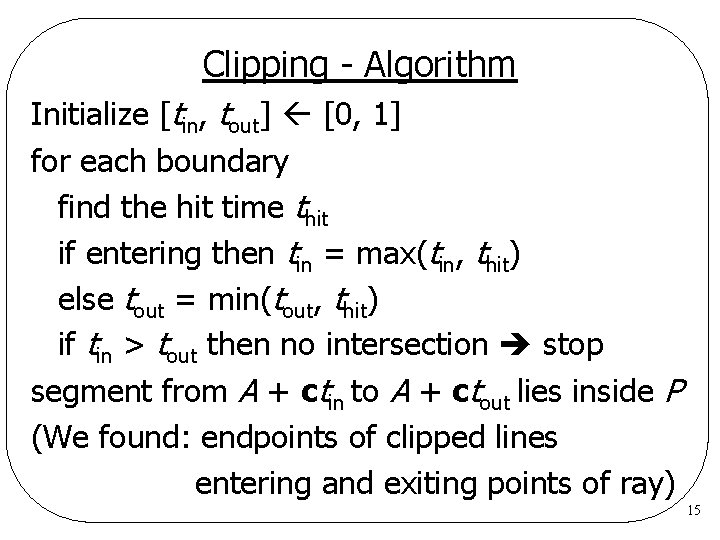Clipping - Algorithm Initialize [tin, tout] [0, 1] for each boundary find the hit time thit if entering then tin = max(tin, thit) else tout = min(tout, thit) if tin > tout then no intersection stop segment from A + ctin to A + ctout lies inside P (We found: endpoints of clipped lines entering and exiting points of ray) 15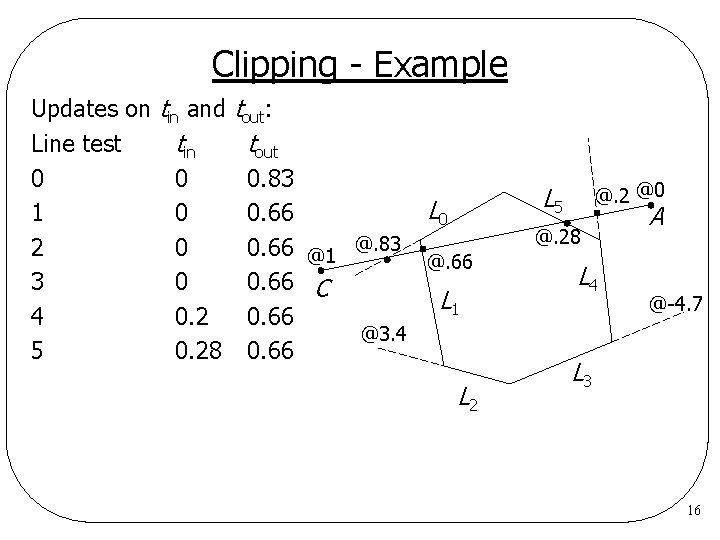Clipping - Example Updates on tin and Line test tin 0 0 1 0 2 0 3 0 4 0. 2 5 0. 28 tout: tout 0. 83 L 0 0. 66 @1 @. 83 @. 66 0. 66 C L 1 0. 66 @3. 4 0. 66 L 2 @0 L 5 @. 28 L 4 A @-4. 7 L 3 16Cyrus-Beck Clipping Algorithm Clip a line segment against any convex polygon l Input parameters l z. Line segment z. List of bounding lines l Output parameter z. Clipped line segment l Return value z 1, if part of segment lies in P z 0, otherwise 17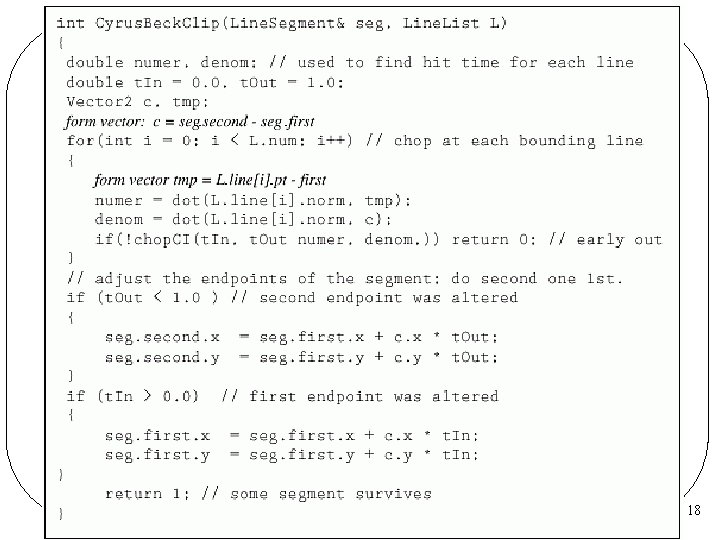1819